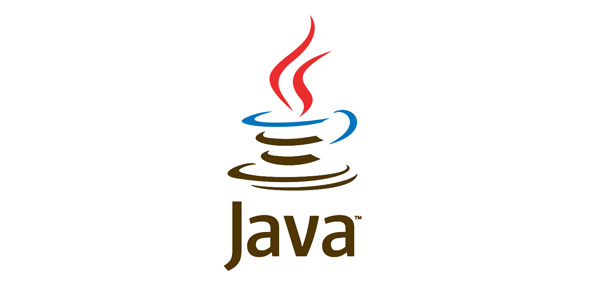# Data Types In Java! Trivia Questions Quiz

10 Questions | Attempts: 109
ShareSettingsBelow is a Data Types in Java Trivia Questions Quiz. There are two data types in java, and this is primitive and non-primitive. The main difference between the two is that one is already defined by java, and the programmer creates the other. Take the quiz and get to refresh your knowledge of the two and how they are applied.

• 1.
Groovy has a literal form for each of its numeric literals except BigDecimal.
• A.

True

• B.

False

• 2.
Following this Groovy declaration, Integer i, the variable i has a fixed, non-changing type.
• A.

True

• B.

False

• 3.
Groovy is like JavaScript in that you can enclose a double quoted string within a single quoted string (e.g. 'Hello "John" ') without escaping each double quote.
• A.

True

• B.

False

• 4.
The find operator =~ returns a Boolean value indicating whether one string is found within another string.
• A.

True

• B.

False

• 5.
What is printed by the following Groovy code?def x = 1/2def y = 1/2println x+y
• A.

0 // since 1/2 uses integer division to produce 0

• B.

1 // since 1/2 + 1/2 = 1

• C.

1.0 // since .5 + .5 = 1.0

• 6.
The subscript read operator a[b] is mapped to
• A.

Get()

• B.

GetAt()

• C.

Index()

• D.

IndexAt()

• 7.
String interpolation performs expression evaluation when the expression following \$ is enclosed in (two words) _____ _____.
• 8.
If you want integer division results in Groovy, you must "escape" to Java coding
• A.

True

• B.

False

• 9.
The 'as type' operator acts like a cast operator.
• A.

True

• B.

False

• 10.
The Number class is augmented in Groovy with the method(s):
• A.

Upto()

• B.

Downto()

• C.

Step()

• D.

All of the above

## Related TopicsBack to top
×

Wait!
Here's an interesting quiz for you.